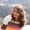Lessons: 20Length: 1.7 hours• Overview
• Transcript

# 3.6 Loops and Iterators: the while and do...while Loops

In this lesson, I'll explain how to use the while and do...while loops. Along the way, you'll see some examples of each and learn the difference between the two.

## 5.Conclusion1 lesson, 01:17

### 3.6 Loops and Iterators: the while and do...while Loops

In this lesson we will be learning about the while and while do loop. If you haven't followed the lessons introduction to loops and iterators and the for loop please go an have a look, because it is important you're familiar with the concepts in there if you want to get yourself clear with this lesson. So let's start implementing the while loop. We will first define a variable here, which will be of int type, and let's assign it the value of 1. And now as the syntax of the while loop, there is the while keyword. And we have a condition check for the counter variable which is i. In the while block we will write the code we want to execute. Keeping it simple, I will print i itself, and then inside the while loop, we need to increment the value of the counter variable. Initially it was 1. The condition is set. 1 is less than 5. It is true. The println statement is executed, or we can say the loop body is executed, and then there is the inclement of the counter variable. Now i changes to 2. Again, it goes to the first line of the while block. 2 less than 5, the statement's condition is true, hence the while block is executed and again, the value of i is increased. Now when the value of i is 5 it will check if 5 is less than 5 no it is false. Because 5 is not less than 5 but it is equal to 5. The loop body will not be executed and it will come out of the while block. And the execution for the while block will be stopped. So now let us run out our code and see what happens. So here we have value of i starting from 1 up to 4. So this is how the while loop works. Now let us change it to 10 and let us print only the even number between the range 1 to 10. And the value of i is increased here and now let us see what happens. Here we have 2, 4, 6 and 8. The even numbers within the range of 1 to 10. So this is all about the while loop. Now let us go to the do while loop. There is a small difference between the while and the do while loop. So what basically happens in do while loop is, if value of i let's say is 11 here the block of code will be executed without the condition being checked and then after the execution of the loop body of at least once then the condition is checked. Now the value of i will be 12, and since it is less than 10, it will come out of the do while loop. I hope you're getting my point. Let's print something here so that we will be able to differentiate between the output of the two functions Okay, so now let us rune our code and see what happens. So here we have 2, 4, 6, 8, which comes from the while loop, and then we have these plain statement the value of i is 11, so that is what it is all about. The block of code is executed at least once, even if the condition is false. Here if we give the value of i as 11, let's see what happens. So here we are in the while loop, this block of code is not executed even once. So this is the basic difference between the while and the do while loop. Let us change it to 1 and see what happens. Here we have the odd number ranging from 1 to 10. i is 1, the loop body is executed. After the increment the condition is changed. And now when the value of i is 10, i is less than 10. It is false, and hence, it doesn't go and execute the loop body again. So this was all about while and do while loop. In the next lesson, we will be learning about the loop control statements, which are break and continue and how do we use this break and continue with the labelled for loop. That's all for this lesson, stay tuned and have a good day.

Back to the top